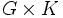# Corollary of Krull-Remak-Schmidt theorem for cancellation of factors in direct product

(diff) ← Older revision | Latest revision (diff) | Newer revision → (diff)
If$G$,$H$, and$K$ are groups of finite composition length such that the external direct products$G \times H$ and$G \times K$ are isomorphic, i.e.,$G \times H \cong G \times K$, then$H \cong K$.